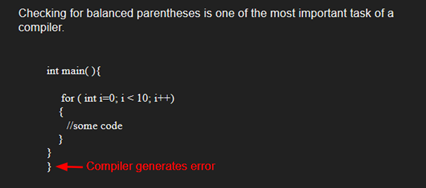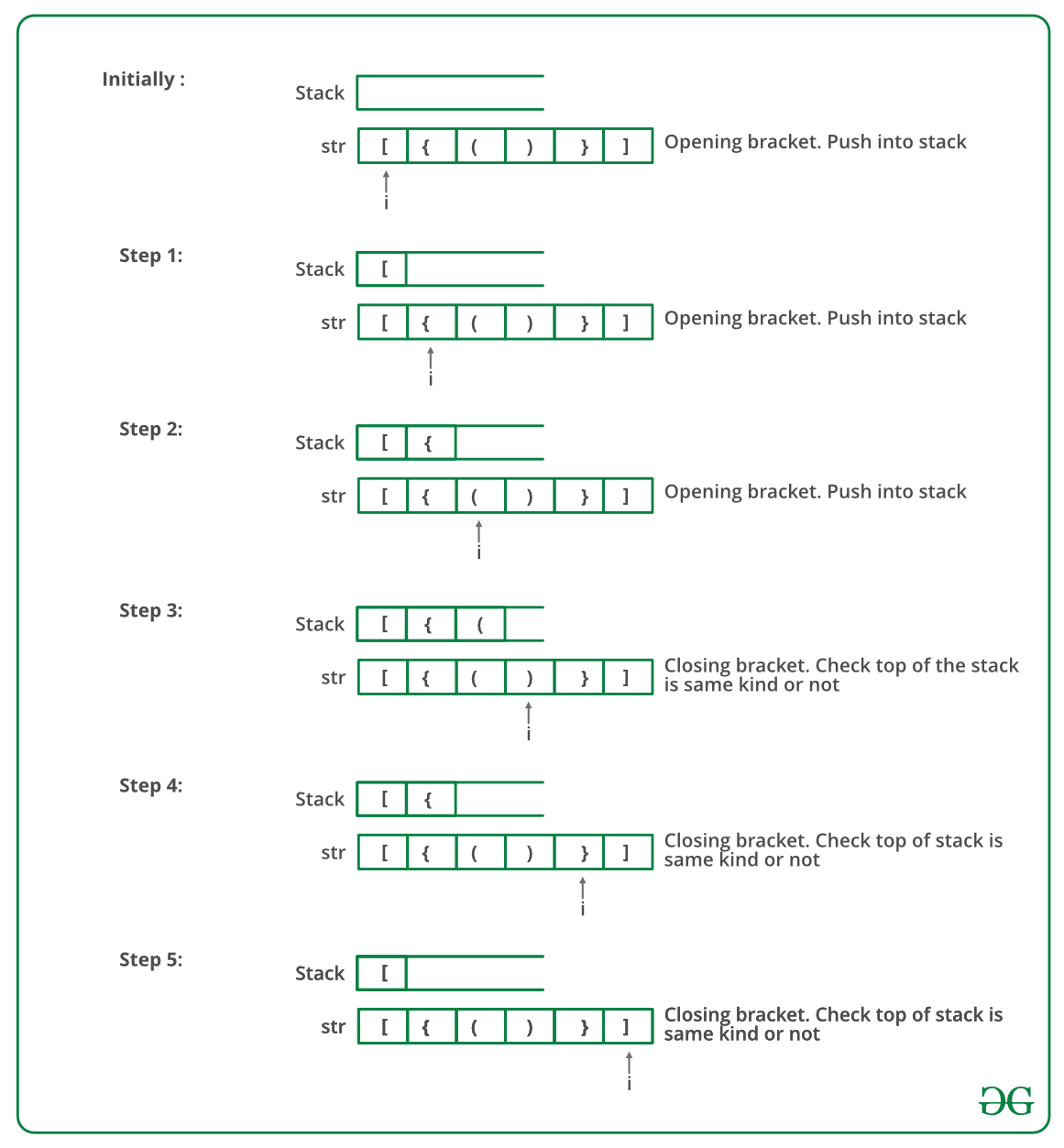Open in App
Not now

# Python Program To Check For Balanced Brackets In An Expression (Well-Formedness) Using Stack

• Last Updated : 19 May, 2022

Given an expression string exp, write a program to examine whether the pairs and the orders of “{“, “}”, “(“, “)”, “[“, “]” are correct in exp.

Example

Input: exp = “[()]{}{[()()]()}”
Output: Balanced

Input: exp = “[(])”
Output: Not BalancedAlgorithm:

• Declare a character stack S.
• Now traverse the expression string exp.
1. If the current character is a starting bracket (‘(‘ or ‘{‘ or ‘[‘) then push it to stack.
2. If the current character is a closing bracket (‘)’ or ‘}’ or ‘]’) then pop from stack and if the popped character is the matching starting bracket then fine else brackets are not balanced.
• After complete traversal, if there is some starting bracket left in stack then “not balanced”

Below image is a dry run of the above approach:Below is the implementation of the above approach:

## Python3

 `# Python3 program to check for ` `# balanced brackets. ` ` `  `# function to check if ` `# brackets are balanced ` ` `  ` `  `def` `areBracketsBalanced(expr): ` `    ``stack ``=` `[] ` ` `  `    ``# Traversing the Expression ` `    ``for` `char ``in` `expr: ` `        ``if` `char ``in` `[``"("``, ``"{"``, ``"["``]: ` ` `  `            ``# Push the element in the stack ` `            ``stack.append(char) ` `        ``else``: ` ` `  `            ``# IF current character is not opening ` `            ``# bracket, then it must be closing. ` `            ``# So stack cannot be empty at this point. ` `            ``if` `not` `stack: ` `                ``return` `False` `            ``current_char ``=` `stack.pop() ` `            ``if` `current_char ``=``=` `'('``: ` `                ``if` `char !``=` `")"``: ` `                    ``return` `False` `            ``if` `current_char ``=``=` `'{'``: ` `                ``if` `char !``=` `"}"``: ` `                    ``return` `False` `            ``if` `current_char ``=``=` `'['``: ` `                ``if` `char !``=` `"]"``: ` `                    ``return` `False` ` `  `    ``# Check Empty Stack ` `    ``if` `stack: ` `        ``return` `False` `    ``return` `True` ` `  ` `  `# Driver Code ` `if` `__name__ ``=``=` `"__main__"``: ` `    ``expr ``=` `"{()}[]"` ` `  `    ``# Function call ` `    ``if` `areBracketsBalanced(expr): ` `        ``print``(``"Balanced"``) ` `    ``else``: ` `        ``print``(``"Not Balanced"``) ` ` `  `# This code is contributed by AnkitRai01 and improved ` `# by Raju Pitta `

Output

`Balanced`

Time Complexity: O(n)
Auxiliary Space: O(n) for stack.

Please refer complete article on Check for Balanced Brackets in an expression (well-formedness) using Stack for more details!

My Personal Notes arrow_drop_up
Related Articles